# 2 6 Rates Ratios and Proportions 2 6

• Slides: 282 -6 Rates, Ratios, and. Proportions 2 -6 Rates, Warm Up Lesson Presentation Lesson Quiz Holt Algebra 1 1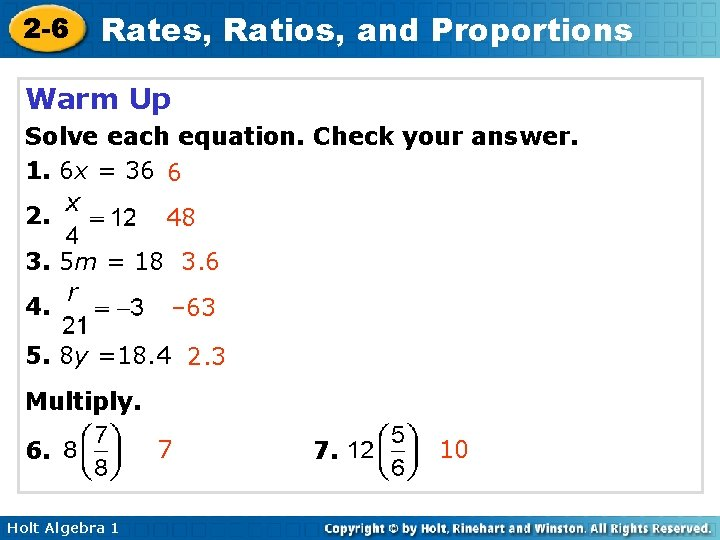2 -6 Rates, Ratios, and Proportions Warm Up Solve each equation. Check your answer. 1. 6 x = 36 6 2. 48 3. 5 m = 18 3. 6 4. – 63 5. 8 y =18. 4 2. 3 Multiply. 6. Holt Algebra 1 7 7. 102 -6 Rates, Ratios, and Proportions Objectives Write and use ratios, rates, and unit rates. Write and solve proportions. Holt Algebra 1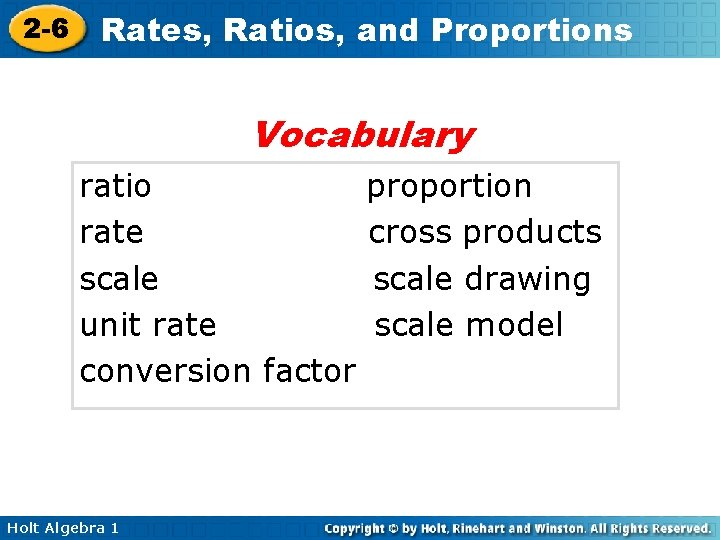2 -6 Rates, Ratios, and Proportions Vocabulary ratio rate scale unit rate conversion factor Holt Algebra 1 proportion cross products scale drawing scale model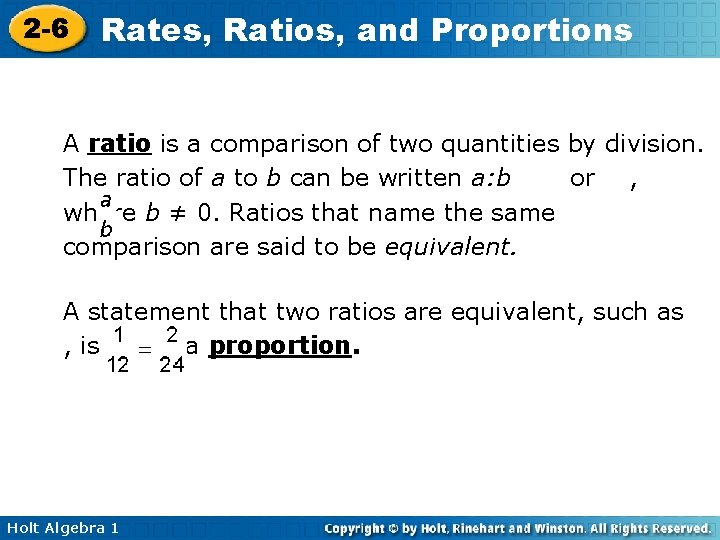2 -6 Rates, Ratios, and Proportions A ratio is a comparison of two quantities by division. The ratio of a to b can be written a: b or , where b ≠ 0. Ratios that name the same comparison are said to be equivalent. A statement that two ratios are equivalent, such as , is called a proportion. Holt Algebra 12 -6 Rates, Ratios, and Proportions Reading Math Read the proportion “ 1 is to 15 as x is to 675”. Holt Algebra 1 as2 -6 Rates, Ratios, and Proportions Example 1: Using Ratios The ratio of the number of bones in a human’s ears to the number of bones in the skull is 3: 11. There are 22 bones in the skull. How many bones are in the ears? Write a ratio comparing bones in ears to bones in skull. Write a proportion. Let x be the number of bones in ears. Since x is divided by 22, multiply both sides of the equation by 22. There are 6 bones in the ears. Holt Algebra 1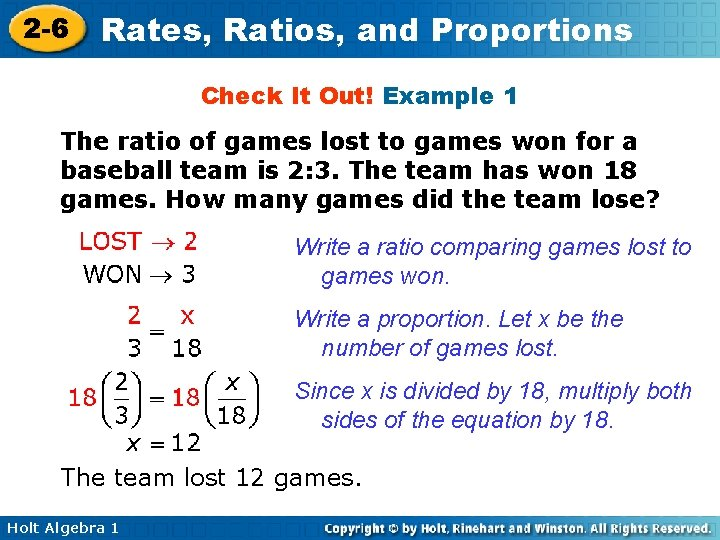2 -6 Rates, Ratios, and Proportions Check It Out! Example 1 The ratio of games lost to games won for a baseball team is 2: 3. The team has won 18 games. How many games did the team lose? Write a ratio comparing games lost to games won. Write a proportion. Let x be the number of games lost. Since x is divided by 18, multiply both sides of the equation by 18. The team lost 12 games. Holt Algebra 12 -6 Rates, Ratios, and Proportions A rate is a ratio of two quantities with different units, such as Rates are usually written as unit rates. A unit rate is a rate with a second quantity of 1 unit, such as or 17 mi/gal. You can convert any rate to a unit rate. Holt Algebra 1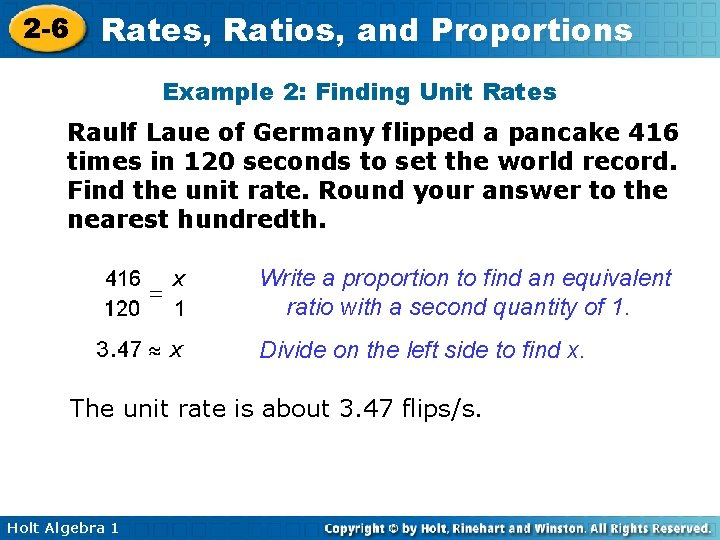2 -6 Rates, Ratios, and Proportions Example 2: Finding Unit Rates Raulf Laue of Germany flipped a pancake 416 times in 120 seconds to set the world record. Find the unit rate. Round your answer to the nearest hundredth. Write a proportion to find an equivalent ratio with a second quantity of 1. Divide on the left side to find x. The unit rate is about 3. 47 flips/s. Holt Algebra 12 -6 Rates, Ratios, and Proportions Check It Out! Example 2 Cory earns \$52. 50 in 7 hours. Find the unit rate. Write a proportion to find an equivalent ratio with a second quantity of 1. Divide on the left side to find x. The unit rate is \$7. 50. Holt Algebra 1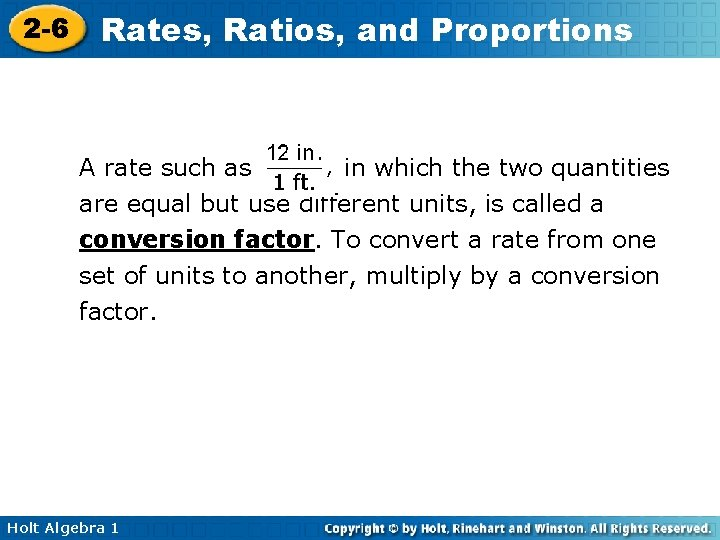2 -6 Rates, Ratios, and Proportions A rate such as in which the two quantities are equal but use different units, is called a conversion factor. To convert a rate from one set of units to another, multiply by a conversion factor. Holt Algebra 12 -6 Rates, Ratios, and Proportions Example 3 A: Converting Rates Serena ran a race at a rate of 10 kilometers per hour. What was her speed in kilometers per minute? Round your answer to the nearest hundredth. To convert the second quantity in a rate, multiply by a conversion factor with that unit in the first quantity. The rate is about 0. 17 kilometer per minute. Holt Algebra 1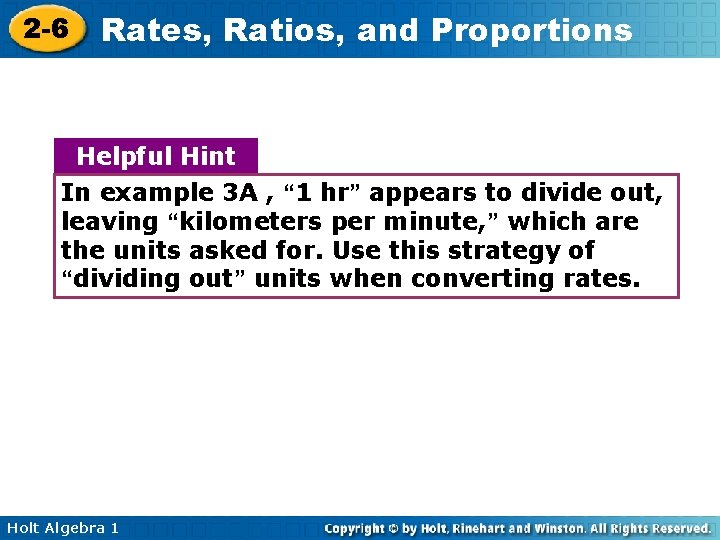2 -6 Rates, Ratios, and Proportions Helpful Hint In example 3 A , “ 1 hr” appears to divide out, leaving “kilometers per minute, ” which are the units asked for. Use this strategy of “dividing out” units when converting rates. Holt Algebra 1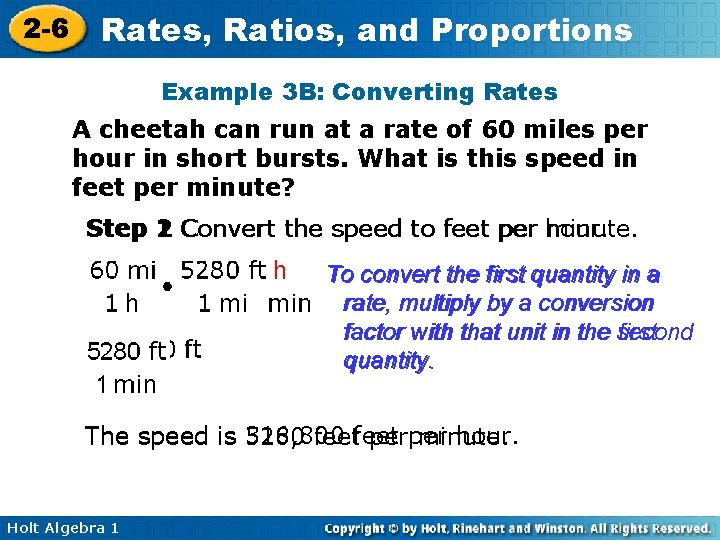2 -6 Rates, Ratios, and Proportions Example 3 B: Converting Rates A cheetah can run at a rate of 60 miles per hour in short bursts. What is this speed in feet per minute? Step 1 2 Convert the speed to feet per hour. minute. To convert the first quantity in a rate, multiply by a conversion factor with that unit in the second first quantity. The speed is 316, 800 hour. 5280 feet perper minute. Holt Algebra 1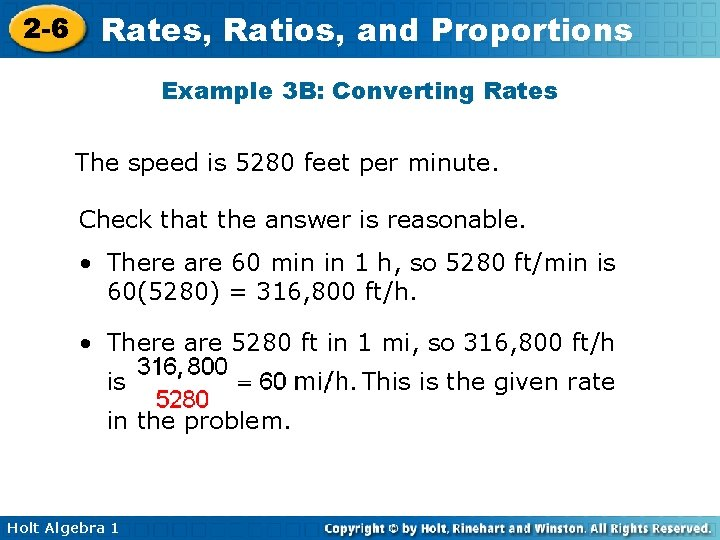2 -6 Rates, Ratios, and Proportions Example 3 B: Converting Rates The speed is 5280 feet per minute. Check that the answer is reasonable. • There are 60 min in 1 h, so 5280 ft/min is 60(5280) = 316, 800 ft/h. • There are 5280 ft in 1 mi, so 316, 800 ft/h is in the problem. Holt Algebra 1 This is the given rate2 -6 Rates, Ratios, and Proportions Check It Out! Example 3 A cyclist travels 56 miles in 4 hours. What is the cyclist’s speed in feet per second? Round your answer to the nearest tenth, and show that your answer is reasonable. Step 1 Convert the speed to feet per hour. Change to miles in 1 hour. To convert the first quantity in a rate, multiply by a conversion factor with that unit in the second quantity. The speed is 73, 920 feet per hour. Holt Algebra 1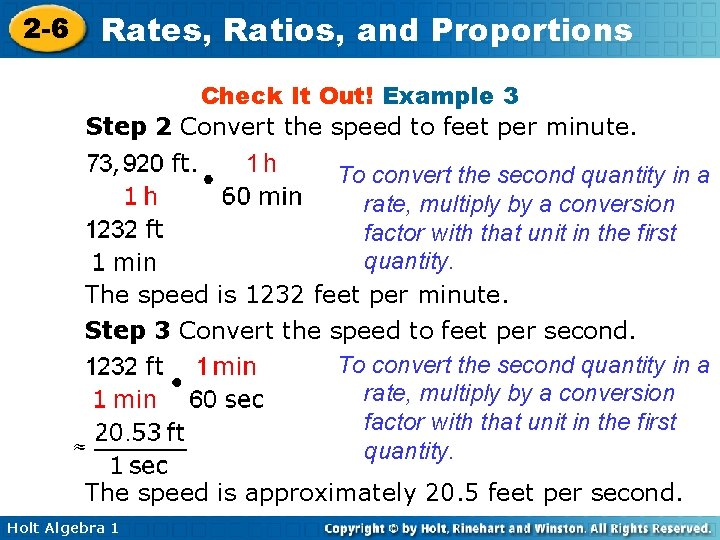2 -6 Rates, Ratios, and Proportions Check It Out! Example 3 Step 2 Convert the speed to feet per minute. To convert the second quantity in a rate, multiply by a conversion factor with that unit in the first quantity. The speed is 1232 feet per minute. Step 3 Convert the speed to feet per second. To convert the second quantity in a rate, multiply by a conversion factor with that unit in the first quantity. The speed is approximately 20. 5 feet per second. Holt Algebra 1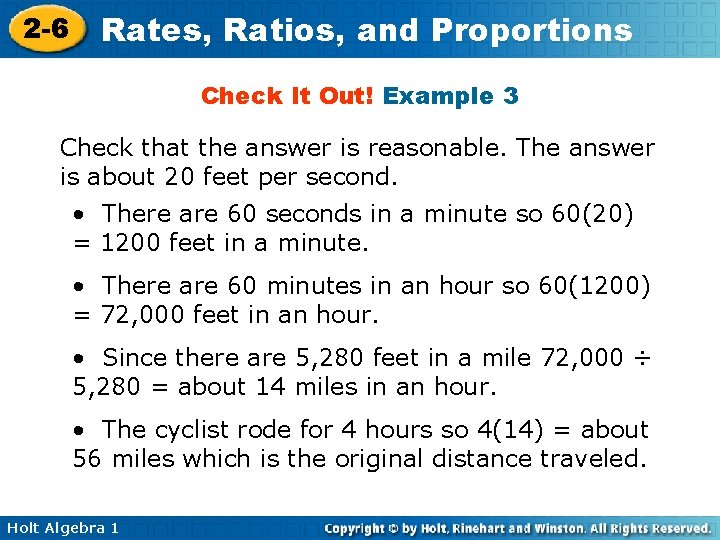2 -6 Rates, Ratios, and Proportions Check It Out! Example 3 Check that the answer is reasonable. The answer is about 20 feet per second. • There are 60 seconds in a minute so 60(20) = 1200 feet in a minute. • There are 60 minutes in an hour so 60(1200) = 72, 000 feet in an hour. • Since there are 5, 280 feet in a mile 72, 000 ÷ 5, 280 = about 14 miles in an hour. • The cyclist rode for 4 hours so 4(14) = about 56 miles which is the original distance traveled. Holt Algebra 12 -6 Rates, Ratios, and Proportions In the proportion , the products a • d and b • c are called cross products. You can solve a proportion for a missing value by using the Cross Products property. Cross Products Property WORDS In a proportion, cross products are equal. Holt Algebra 1 ALGEBRA NUMBERS If 2 • 6=3 • 4 and b ≠ 0 and d ≠ 0 then ad = bc.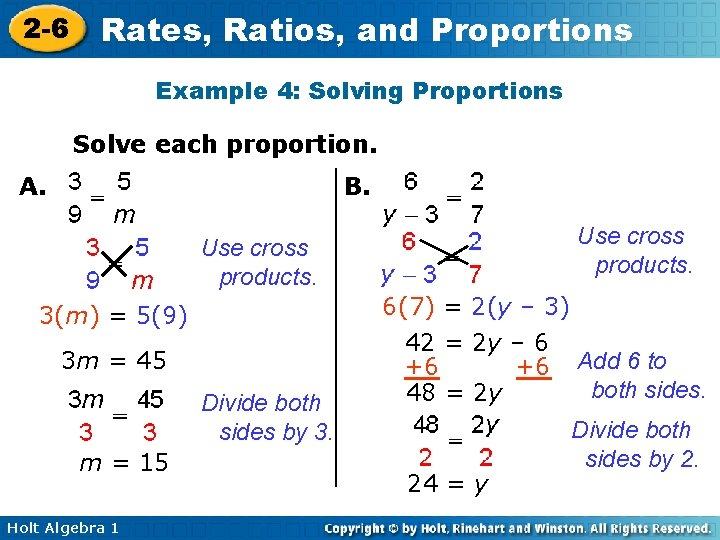2 -6 Rates, Ratios, and Proportions Example 4: Solving Proportions Solve each proportion. A. B. Use cross products. 6(7) = 2(y – 3) 3(m) = 5(9) 3 m = 45 Divide both sides by 3. m = 15 Holt Algebra 1 42 = 2 y – 6 +6 +6 48 = 2 y 24 = y Add 6 to both sides. Divide both sides by 2.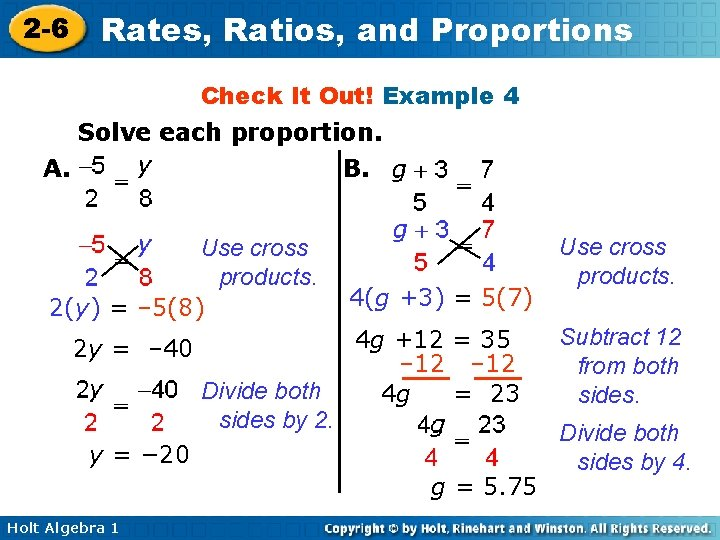2 -6 Rates, Ratios, and Proportions Check It Out! Example 4 Solve each proportion. A. B. Use cross products. 2(y) = – 5(8) 2 y = – 40 Divide both sides by 2. 4(g +3) = 5(7) 4 g +12 = 35 – 12 4 g = 23 y = − 20 g = 5. 75 Holt Algebra 1 Use cross products. Subtract 12 from both sides. Divide both sides by 4.2 -6 Rates, Ratios, and Proportions A scale is a ratio between two sets of measurements, such as 1 in: 5 mi. A scale drawing or scale model uses a scale to represent an object as smaller or larger than the actual object. A map is an example of a scale drawing. Holt Algebra 1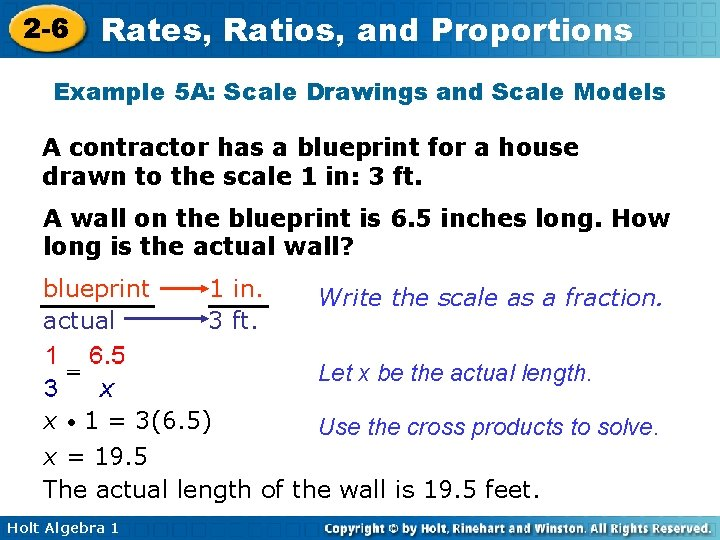2 -6 Rates, Ratios, and Proportions Example 5 A: Scale Drawings and Scale Models A contractor has a blueprint for a house drawn to the scale 1 in: 3 ft. A wall on the blueprint is 6. 5 inches long. How long is the actual wall? blueprint actual 1 in. 3 ft. Write the scale as a fraction. Let x be the actual length. x • 1 = 3(6. 5) Use the cross products to solve. x = 19. 5 The actual length of the wall is 19. 5 feet. Holt Algebra 12 -6 Rates, Ratios, and Proportions Example 5 B: Scale Drawings and Scale Models A contractor has a blueprint for a house drawn to the scale 1 in: 3 ft. One wall of the house will be 12 feet long when it is built. How long is the wall on the blueprint? blueprint actual 1 in. 3 ft. Write the scale as a fraction. Let x be the actual length. 12 = 3 x Use the cross products to solve. Since x is multiplied by 3, divide both sides by 3 to undo the multiplication. 4=x The wall on the blueprint is 4 inches long. Holt Algebra 1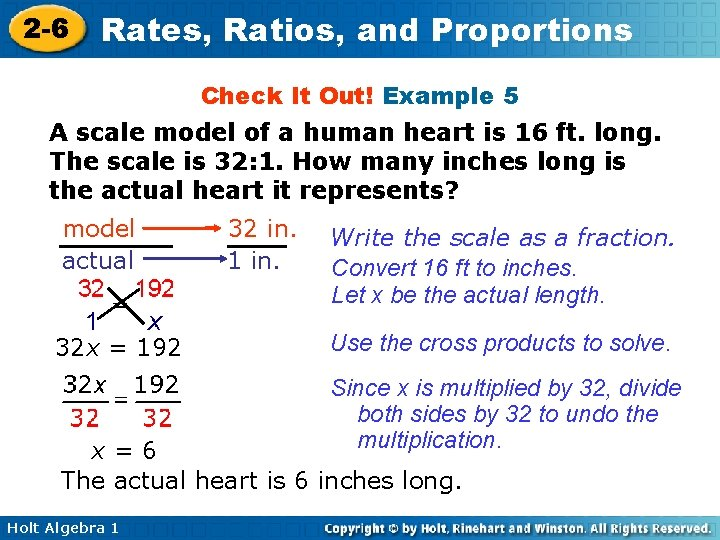2 -6 Rates, Ratios, and Proportions Check It Out! Example 5 A scale model of a human heart is 16 ft. long. The scale is 32: 1. How many inches long is the actual heart it represents? model actual 32 x = 192 32 in. 1 in. Write the scale as a fraction. Convert 16 ft to inches. Let x be the actual length. Use the cross products to solve. Since x is multiplied by 32, divide both sides by 32 to undo the multiplication. x=6 The actual heart is 6 inches long. Holt Algebra 1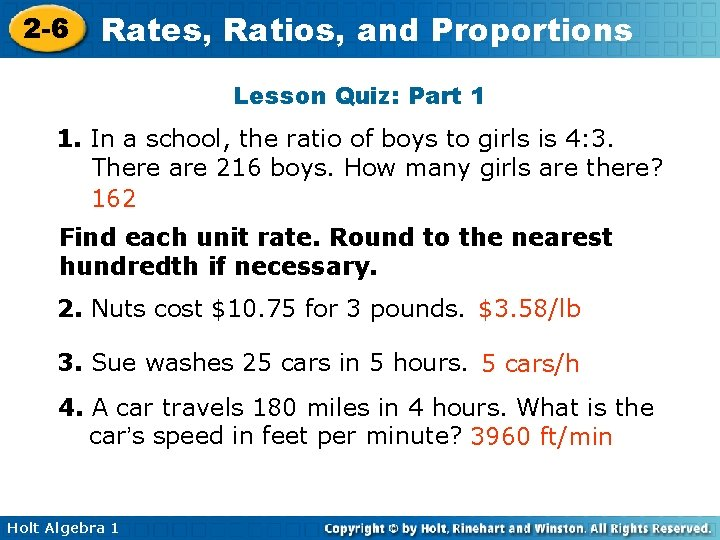2 -6 Rates, Ratios, and Proportions Lesson Quiz: Part 1 1. In a school, the ratio of boys to girls is 4: 3. There are 216 boys. How many girls are there? 162 Find each unit rate. Round to the nearest hundredth if necessary. 2. Nuts cost \$10. 75 for 3 pounds. \$3. 58/lb 3. Sue washes 25 cars in 5 hours. 5 cars/h 4. A car travels 180 miles in 4 hours. What is the car’s speed in feet per minute? 3960 ft/min Holt Algebra 12 -6 Rates, Ratios, and Proportions Lesson Quiz: Part 2 Solve each proportion. 5. 6. 6 16 7. A scale model of a car is 9 inches long. The scale is 1: 18. How many inches long is the car it represents? 162 in. Holt Algebra 1